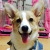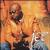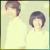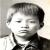## 一个字符串笔试题

crazyinsomnia 发布于 2009/12/23 15:05

0
c

0#### 引用来自“captain_black”的帖子

00
y

0#### 引用来自“yygydjkthh”的帖子

0C++代码：

```int find_substr_num(const TCHAR *str, const TCHAR *substr)
{
int str_len = wcslen(str);
int substr_len = wcslen(substr);

int num_count = 0;
int i = 0;
while(i < str_len)
{
int j = 0;
while(j < substr_len)
{
if ( str[i + j] == substr[j])
j ++;
else
break;
}
if (j >= substr_len)
{
num_count ++;
}
i ++;
}
return num_count;
}```

0#### 引用来自“cghy2011”的答案

C++代码：

```int find_substr_num(const TCHAR *str, const TCHAR *substr)
{
int str_len = wcslen(str);
int substr_len = wcslen(substr);

int num_count = 0;
int i = 0;
while(i < str_len)
{
int j = 0;
while(j < substr_len)
{
if ( str[i + j] == substr[j])
j ++;
else
break;
}
if (j >= substr_len)
{
num_count ++;
}
i ++;
}
return num_count;
}```

0